﻿ Topological insulator. ARPES is wrong

# Massless Dirac fermion is illusion.

## Fictional quasiparticle in quantum mechanics

### [ Quantum mechanics is useless, using unreal concepts. ]

(Fig.1)  Quantum mechanics uses unreal quasiparticles, effective mass.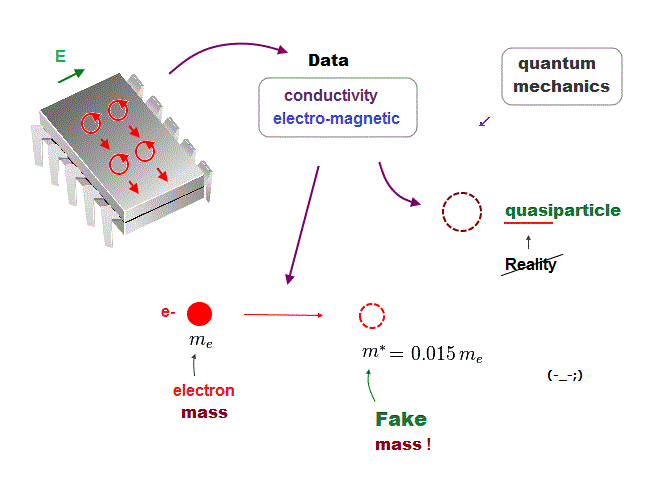The present quantum physics is based on old useless concept 100 years ago, which cannot predict anything.

This is the reason why physicists fabricated fictional targets such as quantum computer and Higgs "symmetry".

In condensed matter physics, useless quantum mechanics completely relies on fictitious quasiparticle and effective mass.

So quantum mechanics clearly prevents us from advancing our science !

## Massless Dirac fermion is unreal.

### [ Top journal and Nobel prize praise fictional particle ? ]

(Fig.2)  Dirac quasiparticle with zero mass doesn't exist !Recently, massless Dirac fermion is a hot topic in solid physics.  But this massless Dirac fermion is just a unreal quasiparticle, which doesn't really exist.

Despite the unreality in massless Dirac quasiparticle, top journal Nature and prestigious Nobel prize ( this p.4 ) applaud it !

This situation is clearly very harmful to students's future and our science.  In fact, this massless Dirac fermion is illusion caused by wrong assumption.

## Massless Dirac quasiparticle is contradictory.

### [ Massless, but it moves slower than light speed c ? ]

(Fig.3)  Quasiparticle contradicts basic physical principle.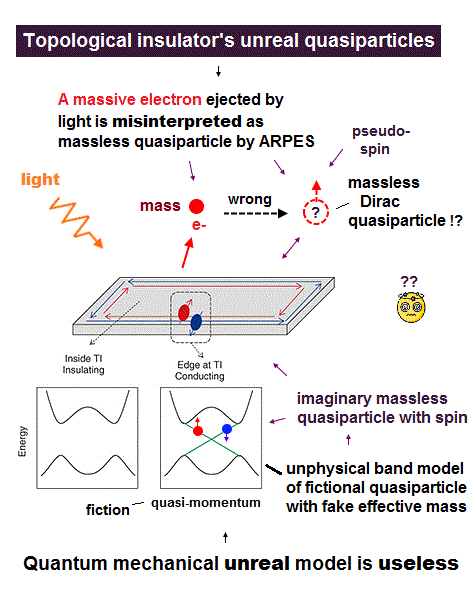Famous Dirac fermion with zero mass is unreal quasiparticle.

When illuminating some material ( ex. topological insulator or graphite ), an electron is ejected by photoelectric effect.

After measuring the energy, momentum of the ejected electron, they jumped to the wrong conclusion that massless Dirac quasiparticle existed inside material.

So they cannot observe this fictitious Dirac quasiparticle, they just detect only the ejected electron.

How fast is this massless (= zero mass ) Dirac fermion moving ?
Einstein relativity claims massless particle always moves at light speed c.

But they say this massless Dirac quasiparticle moves slower than the light speed, 0.3 percent of c, like massless (← ? ) Weyl fermion.

This Dirac quasiparticle contradicts Einstein relativity, so unreal.
Furthermore, it depends on pseudo-spin.

## Angle-Resolved Photoemission Spectroscopy (= ARPES )

### [ Massless Dirac quasiparticle is illusion created by ARPES ! ]

(Fig.4)  Incident photon is absorbed and ejects an electron.How do physicist detect unreal massless Dirac fermion ?
They use Angle-Resolved photoemission Spectroscopy (= ARPES ).

In this ARPES, they illuminate materials with light (= photon ? ) and eject an electron inside solid by photoelectric effect.

So they just measure the "ejected electron" to conclude massless Dirac quasiparticle !

## ARPES depends on wrong assumption !

### [ Electron's momentum inside and outside solid is unchanged !? ]

(Fig.5)  They misunderstand the electron's momentum in ARPES.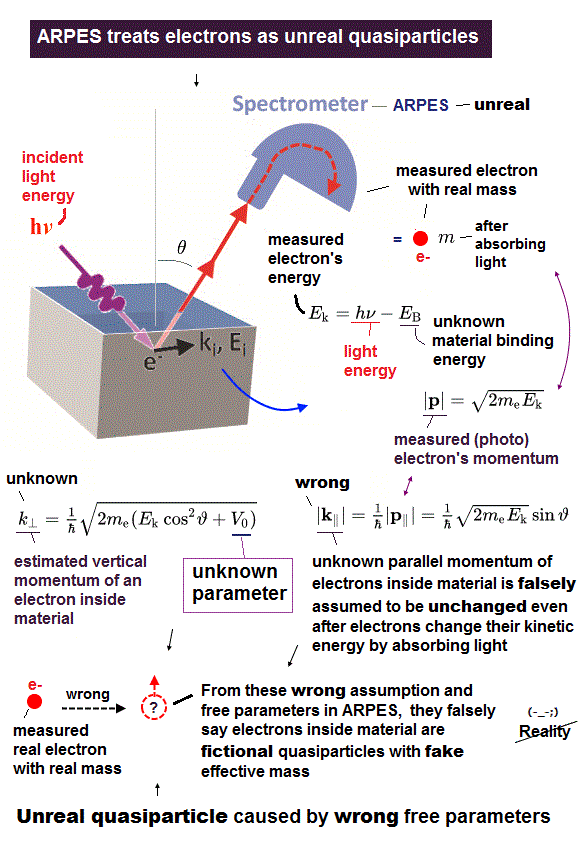In this ARPES, physicists measure the kinetic energy (= Ef ) and momentum (= ħkf ) of the expelled electron by light.

To identify massless Dirac particle, they have to know the relationship between energy and momentum (= ki ) inside the materials.

This is the point where they make serious mistakes.

They jump to the wrong assumption that electron's momentum (= parallel ) doesn't change inside and outside the materials ( this p.27 ) !

## Wrong assumption causes fictional particles !

### [ "Real" electron's momentum "changes" after absorbing light ! ]

(Fig.6)  ARPES assumption = unchanged electron's momentum is false.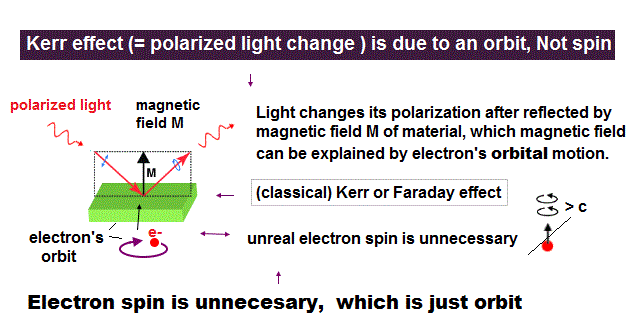To estimate the existence of massless Dirac fermion, they have to know the exact relationship between the kinetic energy and momentum of the electron inside solids.

But all they can do is measure those values only after the electron is ejected by light.  So it's very hard to know the electron's state before absorbing light.

Here, physicists depend on false assumption that the electron's momentum doesn't change before and after the electron absorbs light !

## Conclusion: massless Dirac fermion is illusion

### [ caused by far-fetched interpretation in photoelectron ]

(Fig.7)  Quantum mechanics forces us to fabricate unreal quasiparticle !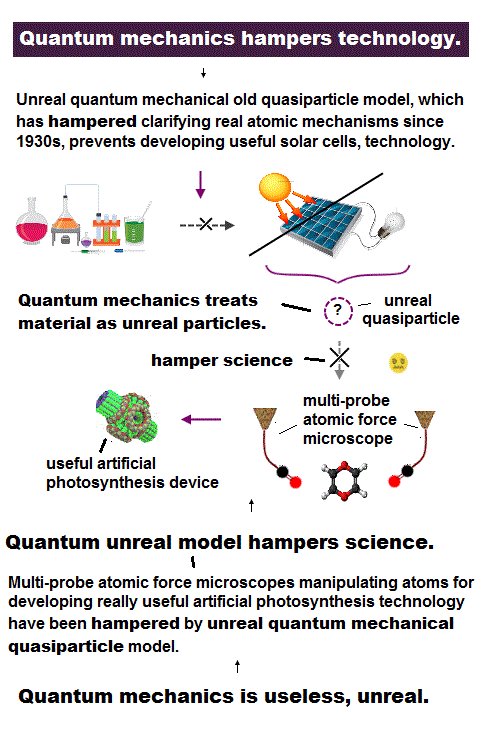The wrong assumption that electron's momentum is unchanged even after absorbing light is a main reason for fictional massless Dirac quasiparticle !

So physicists all over the world waste their time in seeking illusory particles.

## Massless Dirac fermion is detected ?

### [ Energy is linearly proportional to momentum → massless ? ]

(Fig.8)  ARPES wrong assumption about momentum causes "massless".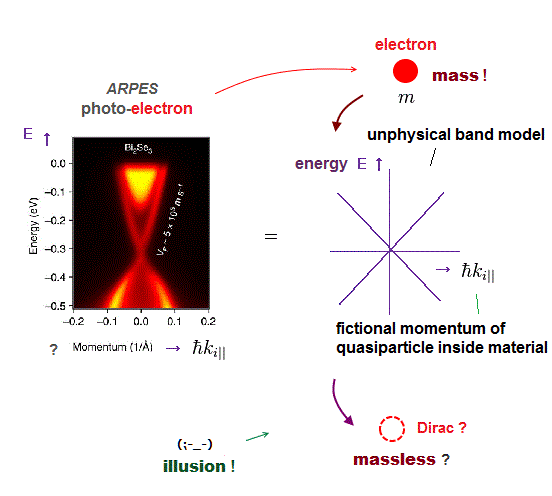Kinetic energy of a particle with mass is proportional to the square of momentum.

On the other hand, the energy of massless light (= photon ) is known to be linearly proportional to momentum ( this p.4 )

By measuring momentum of ejected electron, physicists estimate the relationship of energy linearly proportional to momentum in massless Dirac fermion inside solids.

## Massless Dirac fermion is slower than light !?

### [ "Massless" but slower than the light → unreal quasiparticle. ]

(Fig.9)  Massless Dirac fermion slower than light contradicts relativity !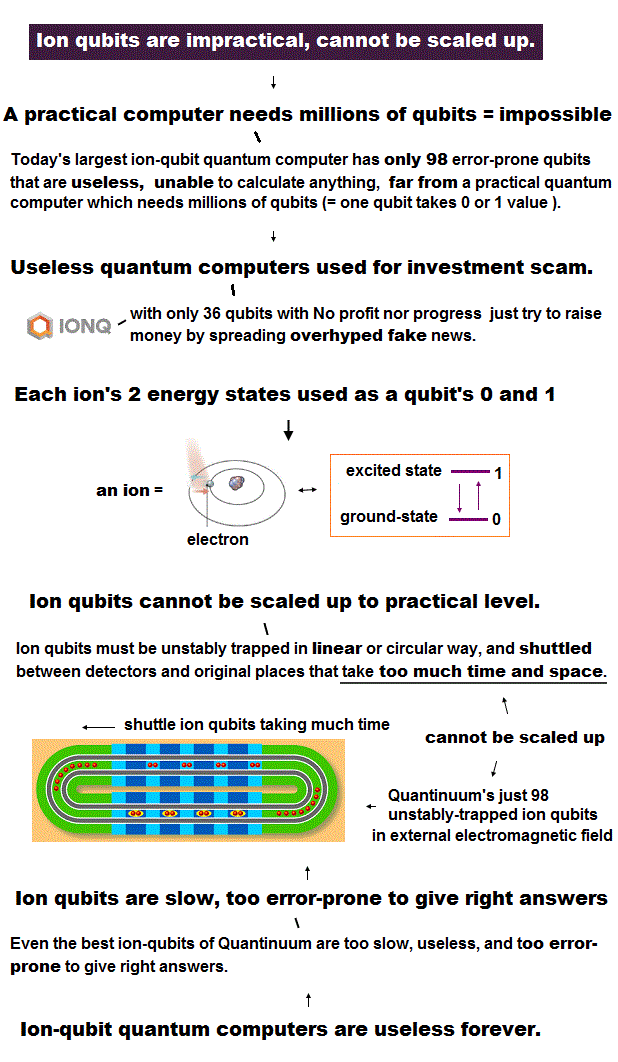Massless particle such as photon must travel at the light speed c in any condition according to Einstein relativity.

Bur surprisingly, this massless Dirac fermion moves 300 times more slowly than the light ( this p.4 ) !

So this massless Dirac particle conradicts Einstein relativity.
This is the reason why it is called unreal quasiparticle.

## Electron is ejected from solid absorbing light.

### [ Total energy of an electron and light is conserved. ]

(Fig.10)  Ejected electron energy = light + initial energy.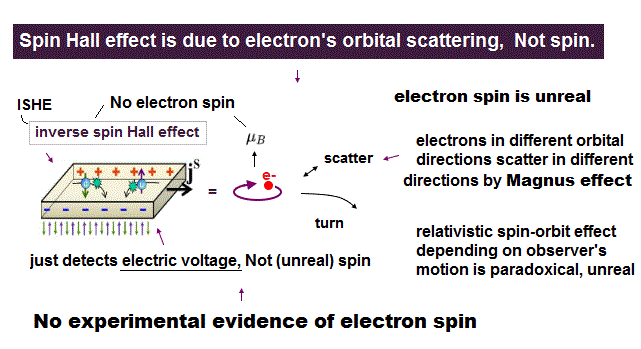Energy of an electron inside the solid is Ei.  After the electron absorbs an incident light with energy (= hν ), their total energy must be conserved.

Final kinetic energy (= Ef ) of the ejected electron is the sum of initial energy and light energy ( this p.27 )

## Electron's momentum inside and outside the solid

### [ Electron momentum before (= pi || ) and after (= pf || ) absorbing light. ]

(Fig.11)  Electron momentum p = ħk.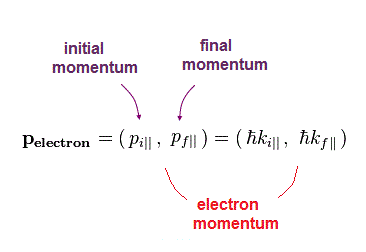Here, electron's momentum inside (= before absorbing light, pi || ) and outside (= after absorbing light, pf || )

## Photon's momentum is zero !?

### [ Wrong assumption = electron momentum doesn't change. ]

(Fig.12)  Then why can an electron increase its kinetic energy ?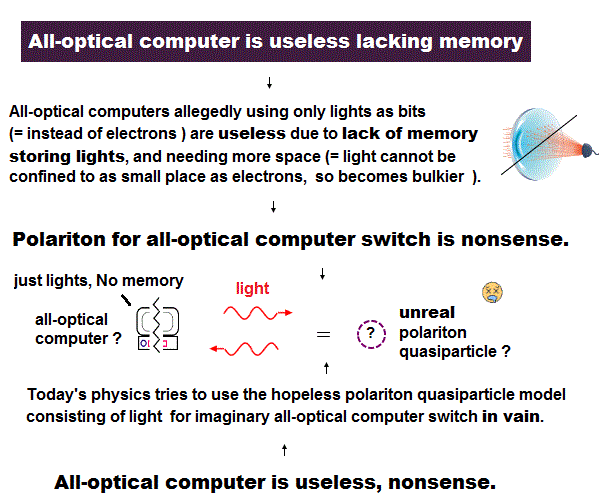Both energy (= E ) and momentum (= p ) must be conserved after an electron absorbs light ( this p.4 ).

Electron's kinetic energy increases by light energy (= hν ).  But they wrongly estimate that the electron's momentum is unchanged even after absorbing light !

Because they think the light has large energy compared to its momentum, so light's momentum can be neglected ( this p.21, this p.2 ).

If photon's momentum is zero and neglected, the ejected electron increases only its kinetic energy without gaining its momentum ?

Unfortunately, it's impossible to increase only electron's kinetic energy without increasing its momentum, because they are proportional to each other.

## The ratio of energy to momentum is different

### [ Heavy electron has larger momentum than the light with the same energy ]

(Fig.13)  Same energy (= E ) → momentum is smaller in photon than electron !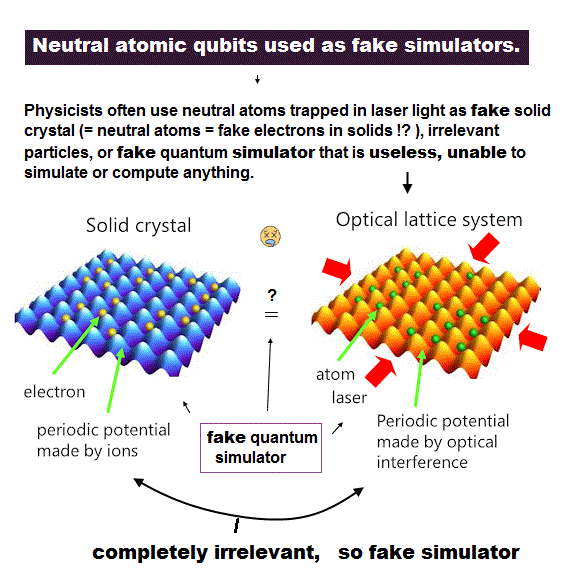Photon (= light ) and an electron clearly have different masses.  The ratios of energy (= E ) to momentum (= p ) are different in particles with different masses.

When an electron and a photon have the same energy (= E ), the momentums of electron and photon are E/(v/2) and E/c, respectively.

Electron's velocity (= v ) is much lower than the light speed c, so, electron's momentum becomes much bigger than that of photon, when their total energies are the same.

## Electron cannot absorb a photon (= light ) !?

### [ Because the ratios of energy to momentum are different. ]

(Fig.14)  So their total momentum is NOT conserved !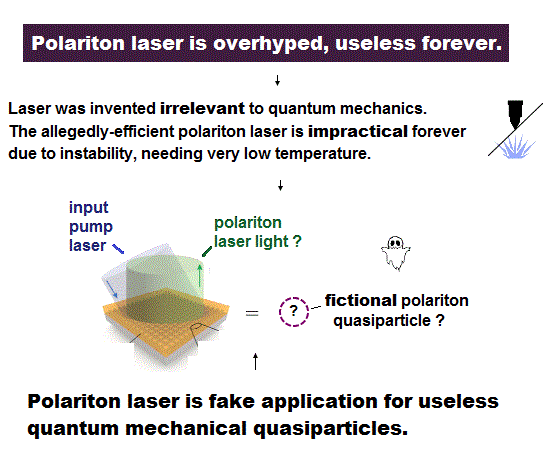If the ratios of energy to momentum in photon and electron are different, it's impossible that an electron absorbs ( or emit ) a photon.

Because both energy and momentum cannot be conserved before and after an electron absorbs light !

It means when an electron absorbs the photon with energy E, their total momentum is NOT conserved !

So we must give up this "photon" model, and adopt more realistic model in an electron absorbing light.

## Light is absorbed into the "whole" atom !

### [ Total momentum of an electron and nucleus remains zero. ]

(Fig.15)  Both an electron and nucleus (= proton ) conserve momentum !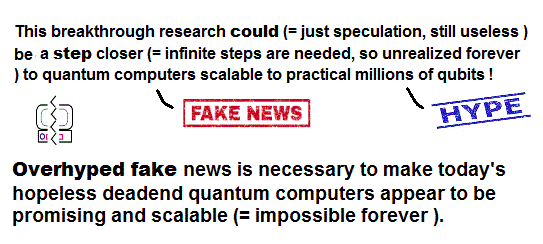Massless Dirac fermion in ARPES depends on wrong assumption, because both momentum and energy cannot be conserved.

If a photon (= light ) is absorbed into the "whole atom" consisting of an electron and a nucleus, this problem doesn't happen.

An eletron and a proton inside atoms are moving in the opposite directions, so have the opposite momentums, and their total momentum is cancelled out to be zero.

Even after the atom absorbs light, their total momentum remains almost zero.  Almost all light energy is transferred only to electron, because a proton is much heavier.

Of course, ejected electron increases not only its kinetic energy but also its momentum ( which is canceled by the opposite proton's momentum ).

So electron's momentum also changes drastically after absorbing light, and we cannot use this assumption !

## Conclusion: massless Dirac quasiparticle is illusion.

### [ They mistakes true electron's momentum for wrong one ! ]

(Fig.16)  Top journal should stop forcing physicistis to seek illusory particle !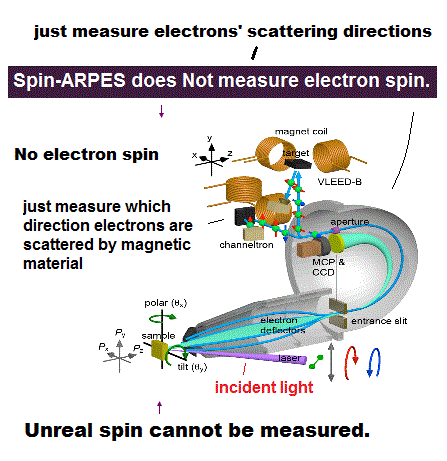As a result, you can easily find that massless Dirac fermion is illusion caused by mistaking true electron's momentum for wrong one.

So all physicists just waste their precious time in illusory particles, encouraged by fraudulent top journals.

Unreal quasiparticle means they are useless forever.  This is the reason why they fabricated imaginary target = quantum computer.2016/5/13 updated. Feel free to link to this site.